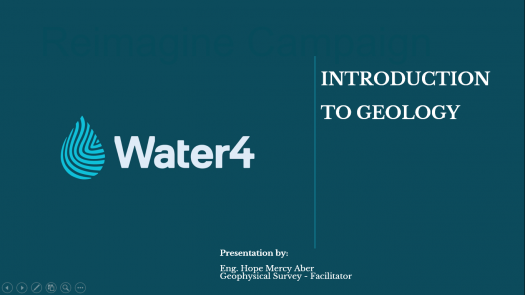# Week 1: Introduction To Geology Quiz

15 Questions | Total Attempts: 45SettingsPAPER CODE: GS 1010 Instructions: There is a total of 15 questions in this paper. The entire paper is divided into two sections, A and B. All sections are compulsory. Questions in each section are of different types. Section – A contains a total of 10 Multiple Choice Questions (MCQ). Each MCQ type question has four choices out of which only one choice is the correct answer. Section – B contains a total of 5 Structured and short assay questions.

• 1.
Referring to the diagram above, path A is _______
• A.

A) Cooling and crystallization

• B.

B) Burial and lithification

• C.

C) Weathering and deposition

• D.

D) Cooling and uplift

• 2.
Referring to the diagram above, path B is _______
• A.

A) Uplift, weathering and erosion, deposition

• B.

B) Deposition, heat and pressure, weathering

• C.

C) Melting, crystallization, heat and pressure

• D.

D) Deposition, lithification and crystallization.

• 3.
Referring to the diagram what factor(s) are responsible for path C?
• A.

A) Melting

• B.

B) Crystallization

• C.

C) Heat and pressure

• D.

D) Burial and lithification

• 4.
One of geology's greatest contributions to human thought is:
• A.

A) The realization that the world is round

• B.

B) The realization that the sun is not the center of the solar system

• C.

C) The recognition of the long span of time involved in earth history

• D.

D) The recognition that the earth is the center of the solar system

• 5.
Because we rarely witness dramatic changes in the Earth, we can conclude that the Earth is not changing very much.
• A.

True

• B.

False

• 6.
Earth materials always trace the complete rock cycle from sediment through igneous rocks.
• A.

True

• B.

False

• 7.
In general, igneous rocks:
• A.

A) Form at relative high temperature

• B.

B) Form by crystallization of solids from liquid

• C.

C) Form at high and low pressures

• D.

D) All of the above

• 8.
Minerals:
• A.

A) Can form by life processes (organic)

• B.

B) Are crystalline solids

• C.

C) Have a unique chemical composition

• D.

D) Can be any state (solid, liquid or gas) as long as the state is natural.

• 9.
Metamorphic rocks are changed rocks. Which of the following rock types could be the "parent" of a metamorphic rock?
• A.

A) Sedimentary

• B.

B) Igneous

• C.

C) Metamorphic

• D.

D) All the above

• 10.
The study of rock deformation and geometry is known as
• A.

A) Structural geology

• B.

B) Geophysics

• C.

C) Engineering geology

• D.

D) Petrology

• 11.
What is geology?
• 12.
Differentiate between a rock and mineral?
• 13.
Name the three types of rocks briefly describing how each is formed?
• 14.
What is weathering?
• 15.
Name three (3) agents of weathering?Back to top